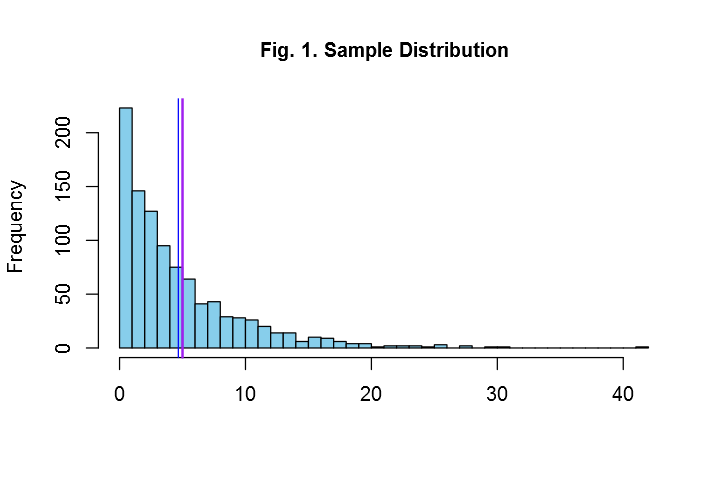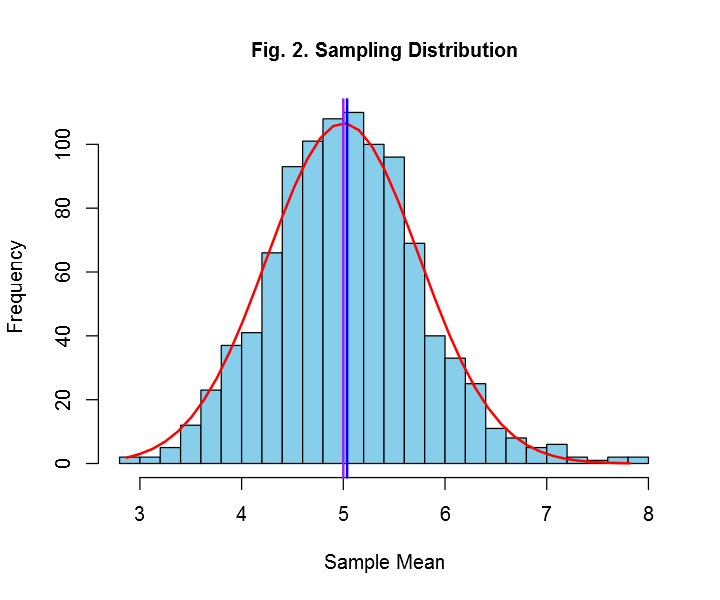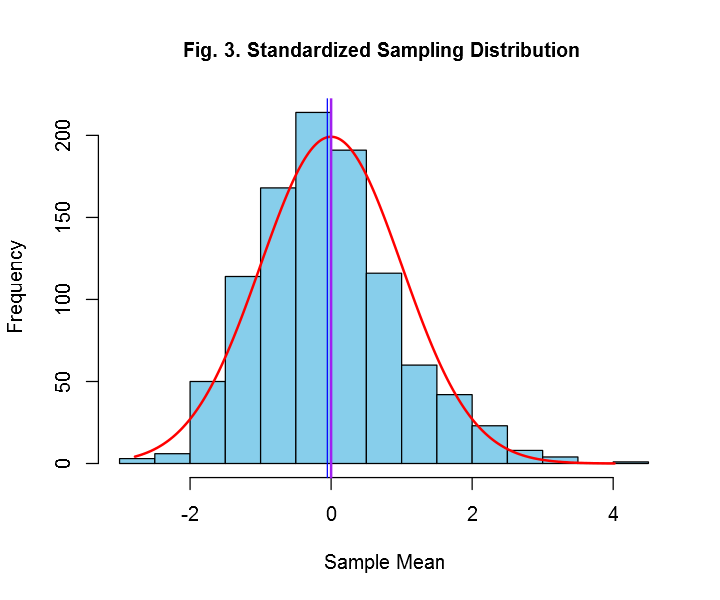# Fisseha Berhane, PhD

#### Data Scientist443-970-2353[email protected] CV Resume### Summary¶

The central limit theorem (CLT) states that the arithmetic mean of a sufficiently large number of iterates of independent random variables, each with a well-defined expected value and well-defined variance, will be approximately normally distributed, regardless of the underlying distribution (Wikipedia). In this project, I demonstrate this theorem using simulations. 40 random samples are drawm from an exponential distribution with mean five, hence standard deviation of five (variance of 25), and their mean is computed. The histogram of 1000 means of 40 random samples (called sampling distribution) is compared with a normal distribution with population mean of five and variance of the product of the square of the standard error of the sampling distribution with the sample size (40 in this case).

### Simulating from Exponential distribution¶

The exponential distribution, which is a particular case of gamma distribution, is a probability distribution that describes the time between events in a Poisson process, i.e. a process in which events occur continuously and independently at a constant average rate (Wikiepdia). Fig. 1 shows exponential distribution with rate of 0.2. The mean and standard deviation of an exponential distribution are the inverse of the rate (lambda) of the distribution, and the variance is the square of 1/$\lambda$. In R to simulate random variables from an exponential distribution with rate of 0.2, we use the command rexp(size, rate=0.2), where 'size' is the number of the random variables to be simulated. In Fig. 1, we have simulated 1000 random variables.

### Simulated mean and variance of an exponential distribution¶

Let's compare the mean and variance of a theoretical exponential distribution with the mean and variance of asimulation.

In :
noquote(paste('Mean of simulation =',round(mean(rexp(1000,rate=0.2)),2)))

noquote(paste('Variance of simulation =',round((sd(rexp(1000,rate=0.2)))^2,2)))

Out:
 Mean of simulation = 5.1
Out:
 Variance of simulation = 25.4

So, we see that the mean and variance are very close to the theoretical mean and variance of an exponential distribution with lambda of 0.2. In Fig. 1, the purple and blue lines are, respectively, the theoretical mean of the exponential distribution with lambda of 0.2 and the mean of the simulation. These two means are very close to each other.

### Sampling Distribution and the CLT¶

Now, let's simulate 40 exponentials 1000 times and draw the histogram of the means of each 40 exponentials and demonstrate the central limit theorem. Fig.2 shows the histogram of the means of 1000 fourty exponentials.

The CLT theorem states that the sampling mean is approximately normally distributed with mean of the population mean (five in this case, because lambda=0.2 and for exponential distribution mean =1/lambda=1/0.2=5) and variance of of the population variance divided by the sample size (40 in our case). In short the sampling distribution is N(μ,σ2/n). Figure 2 clearly demonstartes the CLT theorem because it is bell-shaped and it is approximately centrered at 5. Let's callculate mean and variance of a sampling ditribution of 1000 means from exponenetials of size 40 and compare it with what the CLT tells us.

In :
means = NULL
for (i in 1 : 1000) {means = c(means, mean(rexp(40,rate=0.2)))}

noquote(paste('Mean of sampling distribution =',round(mean(means),2)))

noquote(paste('Variance of sampling distribution =',round(sd(means)^2,2)))

Out:
 Mean of sampling distribution = 5
Out:
 Variance of sampling distribution = 0.59

We see that the mean of the sampling distribution is very close to 5, which is the mean of the exponential distribution with lambda of 0.2, and its variance is very close to 0.625, which is the variance of the exponential distribution (25 for a lambda of 0.2) divided by the sample size (40 in our example). In Fig. 2, the purple and blue lines are, respectively, the theoretical mean, which is 5, and the mean of the sampling distribution. These two means are very close to each other.

We can also compare the sampling distribution with a standard normal (a normal distribution with mean=0 and std=1). Our interest is to check that $\frac{\bar X_n - \mu}{\sigma / \sqrt{n}}$ is approximately a standard normal . Here $\bar X_n$ is the mean of each 40 simulations, $\mu$ is the population mean (five in our case since lambda=0.2 and mean=1/lambda for exponential distribution) and $\sigma$ is the population standard deviation (which is also five for an exponential distribution with lambda=0.2).

Fig. 3. shows a standardized sampling distribution. As the CLT states, we see that it is bell-shaped and cental at about zero (hence a standard normal). Let's calculate mean and variance of a standardized sampling ditribution of 1000 means from exponenetials of size 40 and compare it with what the CLT tells us.

In :
Z = NULL
for (i in 1 : 1000) {

se <-5/sqrt(40)
x= mean(rexp(40,rate=0.2))
x=(x-5)/se
Z = c(Z,x )}

noquote(paste('Mean of standardized sampling distribution =',round(mean(Z),2)))

noquote(paste('Variance of standardized sampling distribution =',round(sd(Z)^2,2)))

Out:
 Mean of standardized sampling distribution = 0.07
Out:
 Variance of standardized sampling distribution = 0.99

So, we see that as CLT states the mean of the standardized sampling distribution is approximately zero and its variance is about one. In Fig. 3, the purple and blue lines are, respectively, the theoretical mean of the standard normal, which is zero, and the mean of the sampling distribution. These two means are very close to each other.

We can also check the normality of the sampling distribution by plotting Q-Q plot as shown in Fig. 4. Since the deviations from the straight line are minimal, we can safely say that Fig.3. is a standard normal distribution.

### Appendix¶

In :
Z= rexp(1000, rate=0.2)
hist(Z, breaks=30, col="skyblue", xlab=" ",
main="Fig. 1. Sample Distribution",cex.main=1)

abline(v=5,col='purple',lwd=2)
abline(v=mean(Z),col='blue')In :
means = NULL

for (i in 1 : 1000) {means = c(means, mean(rexp(40,rate=0.2)))}
h<-hist(means, breaks=20, col="skyblue", xlab="Sample Mean", main="Fig. 2. Sampling Distribution",cex.main=1)

xfit<-seq(min(means),max(means),length=40)
yfit<-dnorm(xfit,mean=5,sd=sd(means)) #sd here is the standard error
yfit <- yfit*diff(h$mids[1:2])*length(means) # getting a multiplier from the hist object lines(xfit, yfit, col="red", lwd=2) abline(v=5,col='purple',lwd=2) abline(v=mean(means),col='blue',lwd=2)In : Z = NULL for (i in 1 : 1000) { se <-5/sqrt(40) x= mean(rexp(40,rate=0.2)) x=(x-5)/se Z = c(Z,x )} h<-hist(Z, breaks=20, col="skyblue", xlab="Sample Mean", main="Fig. 3. Standardized Sampling Distribution ",cex.main=1) xfit<-seq(min(Z),max(Z),length=1000) yfit<-dnorm(xfit,mean=0,sd=sd(Z)) yfit <- yfit*diff(h$mids[1:2])*length(Z)
lines(xfit, yfit, col="red", lwd=2)
abline(v=0,col='purple',lwd=2)
abline(v=mean(Z),col='blue')In :
qqnorm(Z,main="Fig.4. Normal Q-Q Plot",cex.main=0.9); qqline(Z)